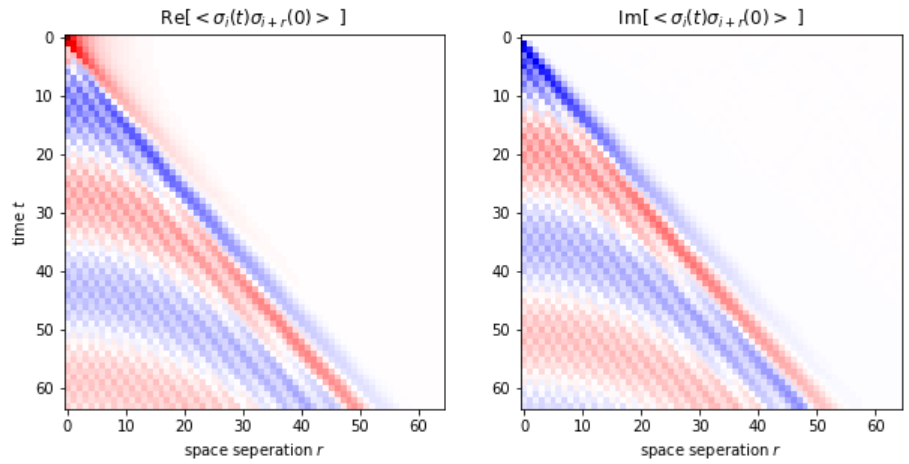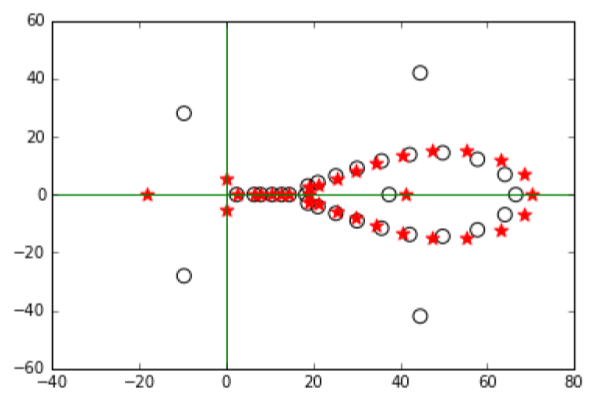﻿ notes

# software package

Computational condensed matter physics.

#### Python package for quantum Ising chainThe general quantum system lattice computation problems are N-P hard.
However, there is a family of spin Chain models which can be mapped to free freemion problem by Jordon-Wigner transformation. The time complexicity to computate a spin-spin correlation function then becomes O(N^3). This software takes the Hamiltonian as input. The output can be spin-spin correlation functions, out-of-time-order correlation functions, spin power spectrum, entanglement entropies, etc..
code   paper

#### Python package for N dimensional Ising model with long range interactions

Ising model still does not have analytical solutions in N>2 dimensions. Numeric simulation plays a crucial rule in understanding the physics. Here, is my software for arbitary dimensions Ising model. Long range interaction feature is added.
code

#### MATLAB package for Rational function (Pade) regression method for numeric analytical continuationAnalytic continuation is one of the bottlenect problems in quantum Monte Carlo simulation.
I have developed a simple method for numerical analytic continuation is developed. It is designed to analytically continue the imaginary time (Matsubara frequency) quantum Monte Carlo simulation results to the real time (real frequency) domain. Such a method is based on the Pade approximation. We modify it to be a linear regression problem, and then use bootstrapping statistics to get the averaged result and estimate the error. Unlike maximum entropy method, no prior information is needed. Test-cases have shown that the spectrum is recovered for inputs with relative error as high as 1%

code   paper

#### PPAP (Python Package for Assessing the Pfaffian)A Pfaffian is the square root of a determinant.
Given a 2N by 2N skew-symmetric matrix. The brute force method will take O((2N - 1)!!) time to compute. However, like her syster determinant, Pfaffian also has a O(N^3) method.
This code implement an iteration algorithm from Xun Jia's paper in 2006 (or see the Appendix B of my paper in 2018).
Wimmer also wrote his Pfaffian packages in 2011 using Householder transformations and Givens rotation. However, our iteration algorithm is much simpler to understand, the benchmarking test shows similar perfromance.

code

About the author: Jian Wang(王健), is a PhD student in theoretical condensed matter physics, in UCLA.